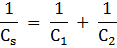### Sample Problem

In the following circuit, what is the value of V1? V.#### Solution

Since C = Q/V, V = Q/C= 1/2 + 1/4 = 3/4

Cs = 4/3 = 1.33 F

C = Q/V

Qtotal = CV = 1.33 F × 80.0 V = 106 C

V = 106 C ÷ 4.00 F = 26.5 V